# sklearn.linear_model.LogisticRegression¶

class sklearn.linear_model.LogisticRegression(penalty='l2', *, dual=False, tol=0.0001, C=1.0, fit_intercept=True, intercept_scaling=1, class_weight=None, random_state=None, solver='lbfgs', max_iter=100, multi_class='auto', verbose=0, warm_start=False, n_jobs=None, l1_ratio=None)[source]

Logistic Regression (aka logit, MaxEnt) classifier.

In the multiclass case, the training algorithm uses the one-vs-rest (OvR) scheme if the ‘multi_class’ option is set to ‘ovr’, and uses the cross-entropy loss if the ‘multi_class’ option is set to ‘multinomial’. (Currently the ‘multinomial’ option is supported only by the ‘lbfgs’, ‘sag’, ‘saga’ and ‘newton-cg’ solvers.)

This class implements regularized logistic regression using the ‘liblinear’ library, ‘newton-cg’, ‘sag’, ‘saga’ and ‘lbfgs’ solvers. Note that regularization is applied by default. It can handle both dense and sparse input. Use C-ordered arrays or CSR matrices containing 64-bit floats for optimal performance; any other input format will be converted (and copied).

The ‘newton-cg’, ‘sag’, and ‘lbfgs’ solvers support only L2 regularization with primal formulation, or no regularization. The ‘liblinear’ solver supports both L1 and L2 regularization, with a dual formulation only for the L2 penalty. The Elastic-Net regularization is only supported by the ‘saga’ solver.

Read more in the User Guide.

Parameters:
penalty{‘l1’, ‘l2’, ‘elasticnet’, None}, default=’l2’

Specify the norm of the penalty:

• None: no penalty is added;

• 'l2': add a L2 penalty term and it is the default choice;

• 'l1': add a L1 penalty term;

• 'elasticnet': both L1 and L2 penalty terms are added.

Warning

Some penalties may not work with some solvers. See the parameter solver below, to know the compatibility between the penalty and solver.

New in version 0.19: l1 penalty with SAGA solver (allowing ‘multinomial’ + L1)

Deprecated since version 1.2: The ‘none’ option was deprecated in version 1.2, and will be removed in 1.4. Use None instead.

dualbool, default=False

Dual (constrained) or primal (regularized, see also this equation) formulation. Dual formulation is only implemented for l2 penalty with liblinear solver. Prefer dual=False when n_samples > n_features.

tolfloat, default=1e-4

Tolerance for stopping criteria.

Cfloat, default=1.0

Inverse of regularization strength; must be a positive float. Like in support vector machines, smaller values specify stronger regularization.

fit_interceptbool, default=True

Specifies if a constant (a.k.a. bias or intercept) should be added to the decision function.

intercept_scalingfloat, default=1

Useful only when the solver ‘liblinear’ is used and self.fit_intercept is set to True. In this case, x becomes [x, self.intercept_scaling], i.e. a “synthetic” feature with constant value equal to intercept_scaling is appended to the instance vector. The intercept becomes intercept_scaling * synthetic_feature_weight.

Note! the synthetic feature weight is subject to l1/l2 regularization as all other features. To lessen the effect of regularization on synthetic feature weight (and therefore on the intercept) intercept_scaling has to be increased.

class_weightdict or ‘balanced’, default=None

Weights associated with classes in the form {class_label: weight}. If not given, all classes are supposed to have weight one.

The “balanced” mode uses the values of y to automatically adjust weights inversely proportional to class frequencies in the input data as n_samples / (n_classes * np.bincount(y)).

Note that these weights will be multiplied with sample_weight (passed through the fit method) if sample_weight is specified.

New in version 0.17: class_weight=’balanced’

random_stateint, RandomState instance, default=None

Used when solver == ‘sag’, ‘saga’ or ‘liblinear’ to shuffle the data. See Glossary for details.

solver{‘lbfgs’, ‘liblinear’, ‘newton-cg’, ‘newton-cholesky’, ‘sag’, ‘saga’}, default=’lbfgs’

Algorithm to use in the optimization problem. Default is ‘lbfgs’. To choose a solver, you might want to consider the following aspects:

• For small datasets, ‘liblinear’ is a good choice, whereas ‘sag’ and ‘saga’ are faster for large ones;

• For multiclass problems, only ‘newton-cg’, ‘sag’, ‘saga’ and ‘lbfgs’ handle multinomial loss;

• ‘liblinear’ is limited to one-versus-rest schemes.

• ‘newton-cholesky’ is a good choice for n_samples >> n_features, especially with one-hot encoded categorical features with rare categories. Note that it is limited to binary classification and the one-versus-rest reduction for multiclass classification. Be aware that the memory usage of this solver has a quadratic dependency on n_features because it explicitly computes the Hessian matrix.

Warning

The choice of the algorithm depends on the penalty chosen. Supported penalties by solver:

• ‘lbfgs’ - [‘l2’, None]

• ‘liblinear’ - [‘l1’, ‘l2’]

• ‘newton-cg’ - [‘l2’, None]

• ‘newton-cholesky’ - [‘l2’, None]

• ‘sag’ - [‘l2’, None]

• ‘saga’ - [‘elasticnet’, ‘l1’, ‘l2’, None]

Note

‘sag’ and ‘saga’ fast convergence is only guaranteed on features with approximately the same scale. You can preprocess the data with a scaler from sklearn.preprocessing.

Refer to the User Guide for more information regarding LogisticRegression and more specifically the Table summarizing solver/penalty supports.

New in version 0.17: Stochastic Average Gradient descent solver.

New in version 0.19: SAGA solver.

Changed in version 0.22: The default solver changed from ‘liblinear’ to ‘lbfgs’ in 0.22.

New in version 1.2: newton-cholesky solver.

max_iterint, default=100

Maximum number of iterations taken for the solvers to converge.

multi_class{‘auto’, ‘ovr’, ‘multinomial’}, default=’auto’

If the option chosen is ‘ovr’, then a binary problem is fit for each label. For ‘multinomial’ the loss minimised is the multinomial loss fit across the entire probability distribution, even when the data is binary. ‘multinomial’ is unavailable when solver=’liblinear’. ‘auto’ selects ‘ovr’ if the data is binary, or if solver=’liblinear’, and otherwise selects ‘multinomial’.

New in version 0.18: Stochastic Average Gradient descent solver for ‘multinomial’ case.

Changed in version 0.22: Default changed from ‘ovr’ to ‘auto’ in 0.22.

verboseint, default=0

For the liblinear and lbfgs solvers set verbose to any positive number for verbosity.

warm_startbool, default=False

When set to True, reuse the solution of the previous call to fit as initialization, otherwise, just erase the previous solution. Useless for liblinear solver. See the Glossary.

New in version 0.17: warm_start to support lbfgs, newton-cg, sag, saga solvers.

n_jobsint, default=None

Number of CPU cores used when parallelizing over classes if multi_class=’ovr’”. This parameter is ignored when the solver is set to ‘liblinear’ regardless of whether ‘multi_class’ is specified or not. None means 1 unless in a joblib.parallel_backend context. -1 means using all processors. See Glossary for more details.

l1_ratiofloat, default=None

The Elastic-Net mixing parameter, with 0 <= l1_ratio <= 1. Only used if penalty='elasticnet'. Setting l1_ratio=0 is equivalent to using penalty='l2', while setting l1_ratio=1 is equivalent to using penalty='l1'. For 0 < l1_ratio <1, the penalty is a combination of L1 and L2.

Attributes:
classes_ndarray of shape (n_classes, )

A list of class labels known to the classifier.

coef_ndarray of shape (1, n_features) or (n_classes, n_features)

Coefficient of the features in the decision function.

coef_ is of shape (1, n_features) when the given problem is binary. In particular, when multi_class='multinomial', coef_ corresponds to outcome 1 (True) and -coef_ corresponds to outcome 0 (False).

intercept_ndarray of shape (1,) or (n_classes,)

Intercept (a.k.a. bias) added to the decision function.

If fit_intercept is set to False, the intercept is set to zero. intercept_ is of shape (1,) when the given problem is binary. In particular, when multi_class='multinomial', intercept_ corresponds to outcome 1 (True) and -intercept_ corresponds to outcome 0 (False).

n_features_in_int

Number of features seen during fit.

New in version 0.24.

feature_names_in_ndarray of shape (n_features_in_,)

Names of features seen during fit. Defined only when X has feature names that are all strings.

New in version 1.0.

n_iter_ndarray of shape (n_classes,) or (1, )

Actual number of iterations for all classes. If binary or multinomial, it returns only 1 element. For liblinear solver, only the maximum number of iteration across all classes is given.

Changed in version 0.20: In SciPy <= 1.0.0 the number of lbfgs iterations may exceed max_iter. n_iter_ will now report at most max_iter.

SGDClassifier

Incrementally trained logistic regression (when given the parameter loss="log_loss").

LogisticRegressionCV

Logistic regression with built-in cross validation.

Notes

The underlying C implementation uses a random number generator to select features when fitting the model. It is thus not uncommon, to have slightly different results for the same input data. If that happens, try with a smaller tol parameter.

Predict output may not match that of standalone liblinear in certain cases. See differences from liblinear in the narrative documentation.

References

L-BFGS-B – Software for Large-scale Bound-constrained Optimization

Ciyou Zhu, Richard Byrd, Jorge Nocedal and Jose Luis Morales. http://users.iems.northwestern.edu/~nocedal/lbfgsb.html

LIBLINEAR – A Library for Large Linear Classification

https://www.csie.ntu.edu.tw/~cjlin/liblinear/

SAG – Mark Schmidt, Nicolas Le Roux, and Francis Bach

Minimizing Finite Sums with the Stochastic Average Gradient https://hal.inria.fr/hal-00860051/document

SAGA – Defazio, A., Bach F. & Lacoste-Julien S. (2014).

“SAGA: A Fast Incremental Gradient Method With Support for Non-Strongly Convex Composite Objectives”

Hsiang-Fu Yu, Fang-Lan Huang, Chih-Jen Lin (2011). Dual coordinate descent

methods for logistic regression and maximum entropy models. Machine Learning 85(1-2):41-75. https://www.csie.ntu.edu.tw/~cjlin/papers/maxent_dual.pdf

Examples

>>> from sklearn.datasets import load_iris
>>> from sklearn.linear_model import LogisticRegression
>>> clf = LogisticRegression(random_state=0).fit(X, y)
>>> clf.predict(X[:2, :])
array([0, 0])
>>> clf.predict_proba(X[:2, :])
array([[9.8...e-01, 1.8...e-02, 1.4...e-08],
[9.7...e-01, 2.8...e-02, ...e-08]])
>>> clf.score(X, y)
0.97...


Methods

 Predict confidence scores for samples. Convert coefficient matrix to dense array format. fit(X, y[, sample_weight]) Fit the model according to the given training data. Get metadata routing of this object. get_params([deep]) Get parameters for this estimator. Predict class labels for samples in X. Predict logarithm of probability estimates. Probability estimates. score(X, y[, sample_weight]) Return the mean accuracy on the given test data and labels. set_fit_request(*[, sample_weight]) Request metadata passed to the fit method. set_params(**params) Set the parameters of this estimator. set_score_request(*[, sample_weight]) Request metadata passed to the score method. Convert coefficient matrix to sparse format.
decision_function(X)[source]

Predict confidence scores for samples.

The confidence score for a sample is proportional to the signed distance of that sample to the hyperplane.

Parameters:
X{array-like, sparse matrix} of shape (n_samples, n_features)

The data matrix for which we want to get the confidence scores.

Returns:
scoresndarray of shape (n_samples,) or (n_samples, n_classes)

Confidence scores per (n_samples, n_classes) combination. In the binary case, confidence score for self.classes_ where >0 means this class would be predicted.

densify()[source]

Convert coefficient matrix to dense array format.

Converts the coef_ member (back) to a numpy.ndarray. This is the default format of coef_ and is required for fitting, so calling this method is only required on models that have previously been sparsified; otherwise, it is a no-op.

Returns:
self

Fitted estimator.

fit(X, y, sample_weight=None)[source]

Fit the model according to the given training data.

Parameters:
X{array-like, sparse matrix} of shape (n_samples, n_features)

Training vector, where n_samples is the number of samples and n_features is the number of features.

yarray-like of shape (n_samples,)

Target vector relative to X.

sample_weightarray-like of shape (n_samples,) default=None

Array of weights that are assigned to individual samples. If not provided, then each sample is given unit weight.

New in version 0.17: sample_weight support to LogisticRegression.

Returns:
self

Fitted estimator.

Notes

The SAGA solver supports both float64 and float32 bit arrays.

Get metadata routing of this object.

Please check User Guide on how the routing mechanism works.

Returns:

A MetadataRequest encapsulating routing information.

get_params(deep=True)[source]

Get parameters for this estimator.

Parameters:
deepbool, default=True

If True, will return the parameters for this estimator and contained subobjects that are estimators.

Returns:
paramsdict

Parameter names mapped to their values.

predict(X)[source]

Predict class labels for samples in X.

Parameters:
X{array-like, sparse matrix} of shape (n_samples, n_features)

The data matrix for which we want to get the predictions.

Returns:
y_predndarray of shape (n_samples,)

Vector containing the class labels for each sample.

predict_log_proba(X)[source]

Predict logarithm of probability estimates.

The returned estimates for all classes are ordered by the label of classes.

Parameters:
Xarray-like of shape (n_samples, n_features)

Vector to be scored, where n_samples is the number of samples and n_features is the number of features.

Returns:
Tarray-like of shape (n_samples, n_classes)

Returns the log-probability of the sample for each class in the model, where classes are ordered as they are in self.classes_.

predict_proba(X)[source]

Probability estimates.

The returned estimates for all classes are ordered by the label of classes.

For a multi_class problem, if multi_class is set to be “multinomial” the softmax function is used to find the predicted probability of each class. Else use a one-vs-rest approach, i.e. calculate the probability of each class assuming it to be positive using the logistic function. and normalize these values across all the classes.

Parameters:
Xarray-like of shape (n_samples, n_features)

Vector to be scored, where n_samples is the number of samples and n_features is the number of features.

Returns:
Tarray-like of shape (n_samples, n_classes)

Returns the probability of the sample for each class in the model, where classes are ordered as they are in self.classes_.

score(X, y, sample_weight=None)[source]

Return the mean accuracy on the given test data and labels.

In multi-label classification, this is the subset accuracy which is a harsh metric since you require for each sample that each label set be correctly predicted.

Parameters:
Xarray-like of shape (n_samples, n_features)

Test samples.

yarray-like of shape (n_samples,) or (n_samples, n_outputs)

True labels for X.

sample_weightarray-like of shape (n_samples,), default=None

Sample weights.

Returns:
scorefloat

Mean accuracy of self.predict(X) w.r.t. y.

set_fit_request(*, sample_weight: Union[bool, None, str] = '$UNCHANGED$') [source]

Request metadata passed to the fit method.

Note that this method is only relevant if enable_metadata_routing=True (see sklearn.set_config). Please see User Guide on how the routing mechanism works.

The options for each parameter are:

• True: metadata is requested, and passed to fit if provided. The request is ignored if metadata is not provided.

• False: metadata is not requested and the meta-estimator will not pass it to fit.

• None: metadata is not requested, and the meta-estimator will raise an error if the user provides it.

• str: metadata should be passed to the meta-estimator with this given alias instead of the original name.

The default (sklearn.utils.metadata_routing.UNCHANGED) retains the existing request. This allows you to change the request for some parameters and not others.

New in version 1.3.

Note

This method is only relevant if this estimator is used as a sub-estimator of a meta-estimator, e.g. used inside a Pipeline. Otherwise it has no effect.

Parameters:
sample_weightstr, True, False, or None, default=sklearn.utils.metadata_routing.UNCHANGED

Metadata routing for sample_weight parameter in fit.

Returns:
selfobject

The updated object.

set_params(**params)[source]

Set the parameters of this estimator.

The method works on simple estimators as well as on nested objects (such as Pipeline). The latter have parameters of the form <component>__<parameter> so that it’s possible to update each component of a nested object.

Parameters:
**paramsdict

Estimator parameters.

Returns:
selfestimator instance

Estimator instance.

set_score_request(*, sample_weight: Union[bool, None, str] = '$UNCHANGED$') [source]

Request metadata passed to the score method.

Note that this method is only relevant if enable_metadata_routing=True (see sklearn.set_config). Please see User Guide on how the routing mechanism works.

The options for each parameter are:

• True: metadata is requested, and passed to score if provided. The request is ignored if metadata is not provided.

• False: metadata is not requested and the meta-estimator will not pass it to score.

• None: metadata is not requested, and the meta-estimator will raise an error if the user provides it.

• str: metadata should be passed to the meta-estimator with this given alias instead of the original name.

The default (sklearn.utils.metadata_routing.UNCHANGED) retains the existing request. This allows you to change the request for some parameters and not others.

New in version 1.3.

Note

This method is only relevant if this estimator is used as a sub-estimator of a meta-estimator, e.g. used inside a Pipeline. Otherwise it has no effect.

Parameters:
sample_weightstr, True, False, or None, default=sklearn.utils.metadata_routing.UNCHANGED

Metadata routing for sample_weight parameter in score.

Returns:
selfobject

The updated object.

sparsify()[source]

Convert coefficient matrix to sparse format.

Converts the coef_ member to a scipy.sparse matrix, which for L1-regularized models can be much more memory- and storage-efficient than the usual numpy.ndarray representation.

The intercept_ member is not converted.

Returns:
self

Fitted estimator.

Notes

For non-sparse models, i.e. when there are not many zeros in coef_, this may actually increase memory usage, so use this method with care. A rule of thumb is that the number of zero elements, which can be computed with (coef_ == 0).sum(), must be more than 50% for this to provide significant benefits.

After calling this method, further fitting with the partial_fit method (if any) will not work until you call densify.

## Examples using sklearn.linear_model.LogisticRegression¶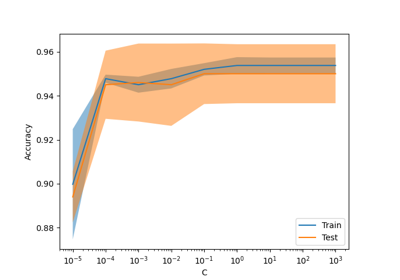Release Highlights for scikit-learn 1.3

Release Highlights for scikit-learn 1.3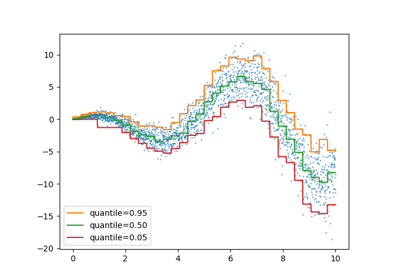Release Highlights for scikit-learn 1.1

Release Highlights for scikit-learn 1.1Release Highlights for scikit-learn 1.0

Release Highlights for scikit-learn 1.0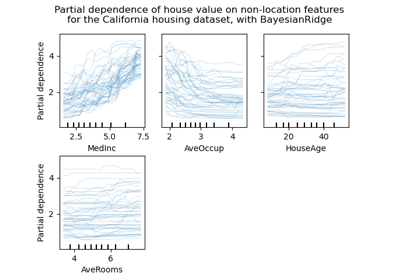Release Highlights for scikit-learn 0.24

Release Highlights for scikit-learn 0.24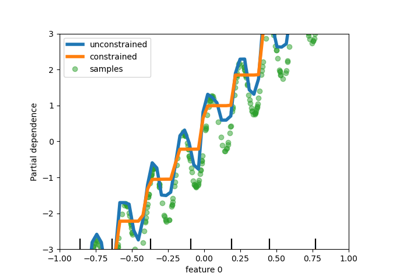Release Highlights for scikit-learn 0.23

Release Highlights for scikit-learn 0.23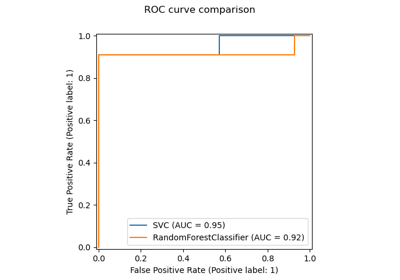Release Highlights for scikit-learn 0.22

Release Highlights for scikit-learn 0.22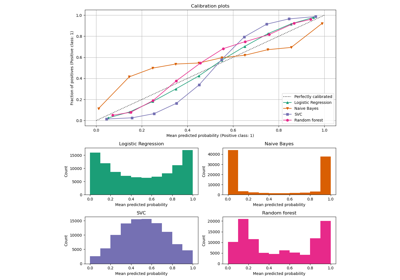Comparison of Calibration of Classifiers

Comparison of Calibration of Classifiers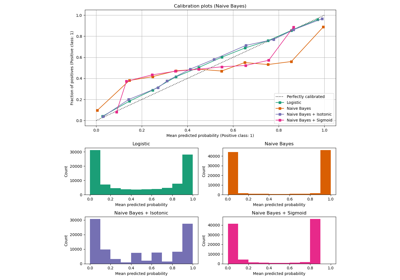Probability Calibration curves

Probability Calibration curves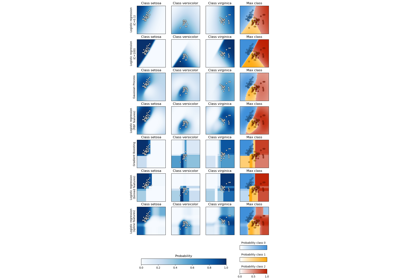Plot classification probability

Plot classification probability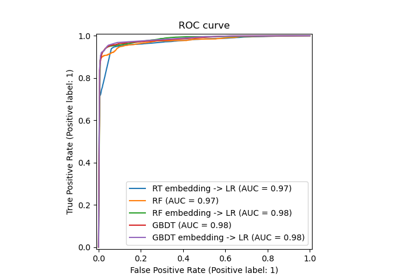Feature transformations with ensembles of trees

Feature transformations with ensembles of treesPlot class probabilities calculated by the VotingClassifier

Plot class probabilities calculated by the VotingClassifier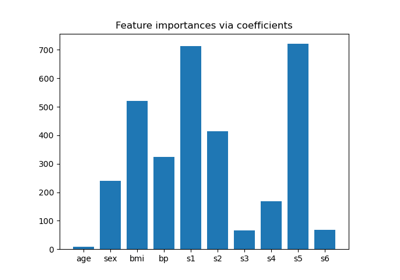Model-based and sequential feature selection

Model-based and sequential feature selection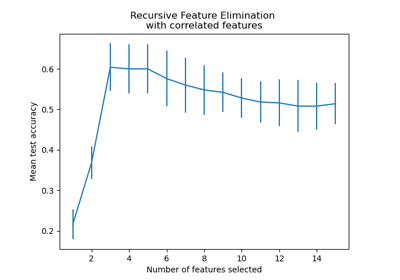Recursive feature elimination with cross-validation

Recursive feature elimination with cross-validationComparing various online solvers

Comparing various online solvers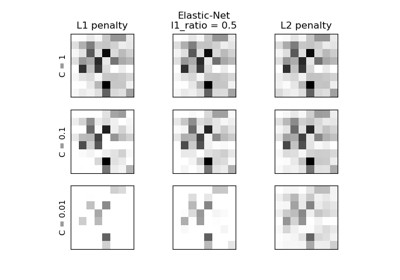L1 Penalty and Sparsity in Logistic Regression

L1 Penalty and Sparsity in Logistic RegressionLogistic Regression 3-class Classifier

Logistic Regression 3-class ClassifierLogistic function

Logistic function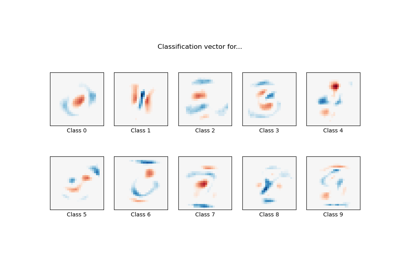MNIST classification using multinomial logistic + L1

MNIST classification using multinomial logistic + L1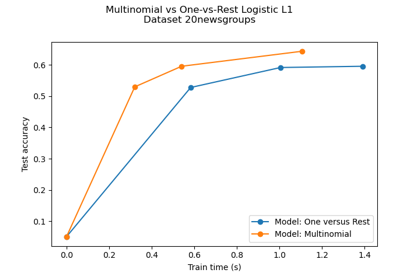Multiclass sparse logistic regression on 20newgroups

Multiclass sparse logistic regression on 20newgroups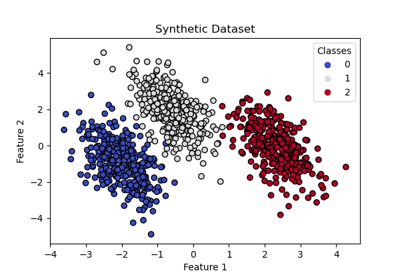Plot multinomial and One-vs-Rest Logistic Regression

Plot multinomial and One-vs-Rest Logistic Regression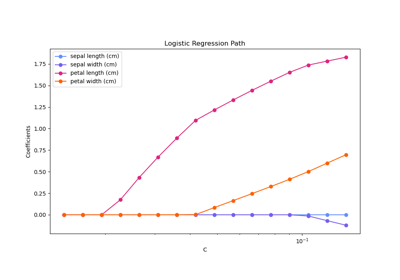Regularization path of L1- Logistic Regression

Regularization path of L1- Logistic RegressionDisplaying Pipelines

Displaying PipelinesDisplaying estimators and complex pipelines

Displaying estimators and complex pipelines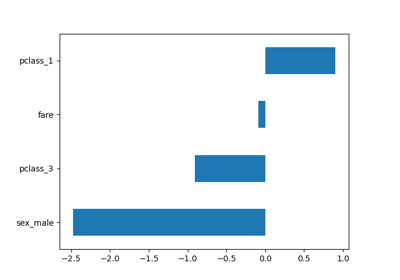Introducing the set_output API

Introducing the set_output API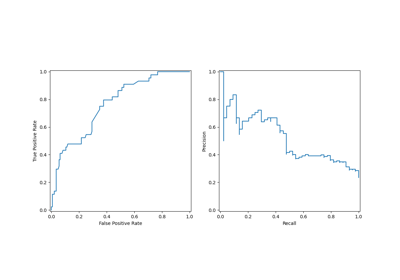Visualizations with Display Objects

Visualizations with Display Objects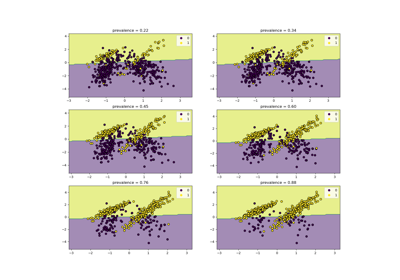Class Likelihood Ratios to measure classification performance

Class Likelihood Ratios to measure classification performance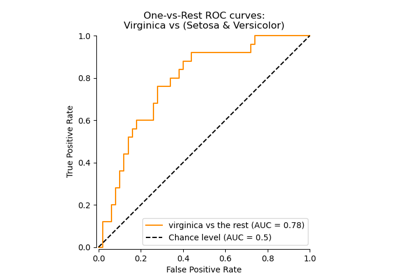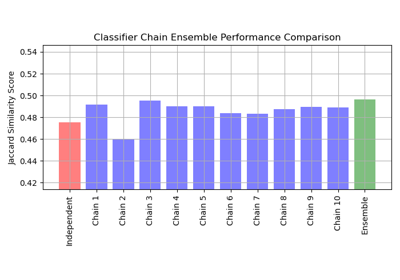Classifier Chain

Classifier Chain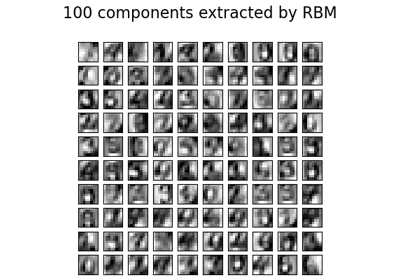Restricted Boltzmann Machine features for digit classification

Restricted Boltzmann Machine features for digit classificationColumn Transformer with Mixed Types

Column Transformer with Mixed Types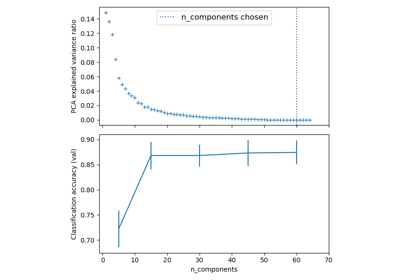Pipelining: chaining a PCA and a logistic regression

Pipelining: chaining a PCA and a logistic regression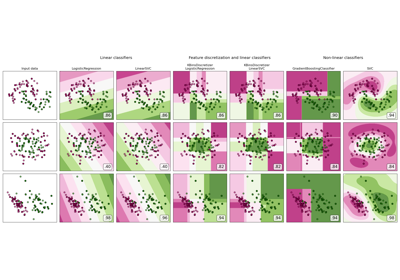Feature discretization

Feature discretizationDigits Classification Exercise

Digits Classification Exercise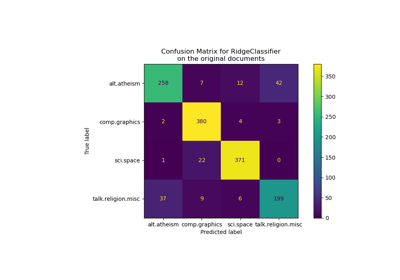Classification of text documents using sparse features

Classification of text documents using sparse features